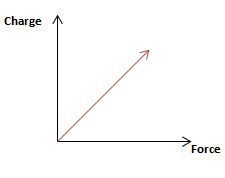# Electric charges

In physics, charge, also known as electric charge, electrical charge, or electrostatic charge and symbolized q, is a characteristic of a unit of matter that expresses the extent to which it has more or fewer electrons than protons. In atoms, the electron carries a negative elementary or unit charge; the proton carries a positive charge. The two types of charge are equal and opposite.

The classical study of electricity is generally divided into three general areas.

#### electrostatics:

the study of the forces acting between charges

#### electric current:

the study of the forms of energy associated with the flow of charge

#### electromagnetism:

the study of the forces acting between charges in motion#### Units

The SI unit of quantity of electric charge is the coulomb, which is equivalent to about 6.242×1018 e (e is the charge of a proton). Hence, the charge of an electron is approximately −1.602×10−19 C.